## July 16, 2004

### Probabilistic STRIPS Operators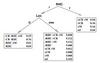From Decision-Theoretic Planning: Structural Assumptions and Computational Leverage:

A classical STRIPS operator is described by a precondition and a set of effects. The former identifies the set of states in which the action can be executed, and the latter describes how the input state changes as a result of taking the action. A probabilistic STRIPS operator (PSO) extends the STRIPS representation in two ways. First, it allows actions to have different effects depending on context, and second, it recognizes that the effects of actions are not always known with certainty.

Formally, a PSO consists of a set of mutually exclusive and exhaustive logical formulae, called contexts, and a stochastic effect associated with each context. Intuitively, a context discriminates situations under which an action can have differing stochastic effects. A stochastic effect is itself a set of change sets -- a simple list of variable values with a probability attached to each change set, with the requirement that these probabilities sum to one. The semantics of a stochastic effect can be described as follows; when the stochastic effect of an action is applied at state s, the possible resulting states are determined by the change sets, each one occurring with the corresponding probability; the resulting state associated with a change set is constructed by changing variable values at state s to match those in the change set, while all unmentioned variables persist in value. Note that since only one context can hold in any state s, the transition distribution for the action at any state s is easily determined.

The context sets can be conveniently represented as a tree which splits on tests of various state variables and whose leaves which are distributions over change sets.

Posted by djp3 at 1:58 PM | Comments (0) | TrackBack (0)

### On the Equivalence of Directed and Undirected Graphical ModelsA directed graphical model can be converted to an undirected graphical model through a process called moralization. Graphically, moralization is accomplished by creating an undirected clique for every node in the directed graph and it's parents.

A directed graph has a conditional probability table (CPT) for every child given its parents. In the undirected graph this CPT becomes the clique potential function, psi. Each node in the undirected graph also has a node potential. If the node had a prior in the directed graph, that becomes the node potential in the undirected graph. Otherwise it is uniformly a constant.

The joint probability function in the directed case goes from:To the undirected case: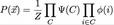Which translates to: the joint probability of a setting of variables, x, is equal to the product of the clique potential functions evaluated with the relevant elements of x passed as parameters, times the node potential functions of all the individual nodes given their queried setting. This has to be scaled by a normalization factor because the original conditional probability tables, while normalized for a given setting of the parent nodes, are not normalized across the joint distribution of parents and child.

Finally it is worth noting that the moralized graph can represent a larger range of probability distributions than the original directed graph. This might be relevant if you desired to learn the parameters of a directed graph. If you did this in a particular way, namely by moralizing the graph and then learning parameters on that graph, the resulting learned graph might not be representable in the original directed graph.

Posted by djp3 at 1:14 PM | Comments (0) | TrackBack (0)

### What is Loopy Belief Propagation?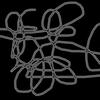Belief propagation is an algorithm for efficiently calculating the probability of a variable in a graphical model given some evidence. This requires marginalizing out all the other variables in the graph. The naive approach to marginalization would lead to an algorithm that is exponential in the size of the graph. Belief propagation is a method which relies on "message passing" to store intermediate sums in the marginalization and can therefore calculate the probability of a variable in time linear in the size of the graph.

Unfortunately, this only leads to exact solutions when the structure of the graph is a tree. If there are loops in the graph then belief propagation becomes "Loopy Belief Propagation." In this case the messages don't have a clear path out and back from the query variable and can be passed in loops. In this case the best one can hope for is that the probability of the query variable converges after lots of message passing. Loopy belief propagation is therefore an approximate solution to a query on a graphical model.

Posted by djp3 at 12:38 PM | Comments (0) | TrackBack (0)

### What exactly is Rao-Blackwellisation?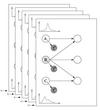From Policy Recognition in the Abstract Hidden Markov Model:
Rao-Blackwellisation is a general technique for improving the accuracy of sampling methods by analytically marginalizing some variables and only sampling the remainder. In its simplest form, consider the problem of estimating the expectation E [f(x)], where x is a joint product of two variables r,z.
Using direct Monte-Carlo sampling, we obtain the estimator: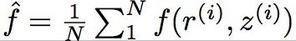Alternatively, a Rao-Blackwellised estimator can be derived by sampling only the variable r, with the other variable z, being integrated out analytically: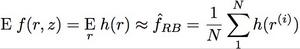where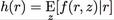.
For our convenience, r will be referred to as the Rao-Blackwellising variable. The Rao-Blackwellised estimator \hat{f}_{RB} is generally more accurate than \hat{f} for the same number of samples N.
Posted by djp3 at 12:10 PM | Comments (0) | TrackBack (0)

## July 15, 2004

### Complexity of Bayesian Network InferenceIt is known that exact inference in Bayesian Networks is NP-Hard with respect to the network size. Cooper, G.F. (1990). The computational complexity of probabilistic inference using Bayesian belief networks. Artificial Intelligence, 42, 393-405 Approximate Bayesian Network inference scales well with network size, but is NP-Hard with respect to the hard-bound accuracy of the estimates. Dagum P., & Luby, M. (1993). Approximating probabilistic inference in Bayesian belief networks is NP-Hard. Artificial Intelligence, 60 141-153.
Posted by djp3 at 5:21 PM | Comments (0) | TrackBack (0)

### Policy Recognition in the Abstract Hidden Markov Model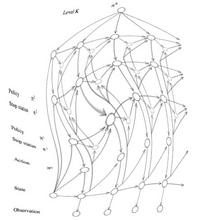This paper seems to be quite an influential paper in the current AI milieu around UW and Intel. In this work, the authors present a method for recognizing policies in an Abstract Hidden Markov Model (AHMM) from a sequence of state observations. The AHMM is an interesting model to try and infer policies on because it is a way of reverse engineering the policy that an Abstract Markov Process (which is a hierarchical version of a Markov Decision Process (MDP)) is executing.

The technique is to compile the AHMM to a DBN and perform inference on the DBN. The authors show that inference on the compiled DBN, which would usually require time exponential in the number of levels in the policy hierarchy, can be achieved in time linear to the size of the policy hierarchy.

They are able to achieve this through a combination of independence assumptions and Rao-Blackwellization. Inference is performed with SIS.

The main contribution of this paper seems to be in showing how to link an MDP to a DBN. If it weren't for this connection, I don't think that the AHMM is really that interesting. The compiled DBN is reasonable to understand and interpret and the presence of the AHMM is unnecessary as a result. In fact in the work that Lin and Dieter did the AHMM was completely unused, although the inference on the DBN borrowed heavily from this paper.

I also wasn't entirely clear on how strong the result was. There was some discussion in the paper about a sub-class of policies in which it was deterministically known that the policy had completed and I didn't follow whether or not that assumption was successfully relaxed or not. Similarly there was an assumption of perfect observability - that the states were known with certainty - that definitely is a difficult restriction to bring into the ubiquitous computing world, but maybe not in the digital world.

Overall it was a very well written paper that provided thorough background and explanation and will likely be a oft-cited paper because of that.

Posted by djp3 at 5:18 PM | Comments (0) | TrackBack (0)

### Understanding Belief Propagation and its Generalizations Part 1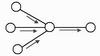This paper did a wonderful job of showing how belief propagation has been applied to a variety of graphical models in a variety of disciplines. It is very clearly written.

The first part of the paper discussed the conversions between and resulting equality of factor graphs, directed graphs and undirected graphs (as well as a few other techniques). Here is a brief summary of the conversion from a directed graph to an undirected graph.

Then the paper proceeded to describe belief propagation on the undirected graphical model. Although the paper didn't mention it, the discussion group that read this paper explained what loopy belief propagation as a consequence. A short summary of that discussion is here.

Posted by djp3 at 10:04 AM | Comments (0) | TrackBack (0)